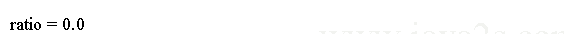# Java Tutorial - Java ternary operator

The `?` operator is a ternary (three-way) operator.

Java ternary operator is basically a short form of simple if statement.

## Syntax

The `?` has this general form:

```expression1 ? expression2 : expression3
```

`expression1` can be any expression that evaluates to a `boolean` value. If `expression1` is `true`, then `expression2` is evaluated. Otherwise, `expression3` is evaluated.

The expression evaluated is the result of the `?` operation. Both `expression2` and `expression3` are required to return the same type, which can't be void.

Here is an example of `?` operator:

```
public class Main {
public static void main(String[] argv) {
int denom = 10;
int num = 4;/* ww w.java2s.  co m*/
double ratio;

ratio = denom == 0 ? 0 : num / denom;
System.out.println("ratio = " + ratio);
}
}
```

The output:## Example

Here is another program that demonstrates the `?` operator. It uses it to obtain the absolute value of a variable.

```
public class Main {
public static void main(String args[]) {
int i, k;//from w  w  w .  j a  va 2s  . c  o  m
i = 10;
k = i < 0 ? -i : i;
System.out.print("Absolute value of ");
System.out.println(i + " is " + k);

i = -10;
k = i < 0 ? -i : i;
System.out.print("Absolute value of ");
System.out.println(i + " is " + k);

}
}
```

The output generated by the program is shown here: